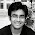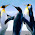## Easiest Shortcut Trick to Solve Clock related Problems in Reasoning for SSC CGL Tier-1 Exam

In this post, I will give you the trick for solving Problems based on Clock. Before you start solving these problems, you must remember below given important points because they will be used.

1. An accurate clock shows 8 o'clock in the morning. Through how many degrees will the hour hand rotate when the clock shows 2 o'clock in the afternoon?
(a) 144                   (b)150
(c) 168                   (d)180
Sol - 8 o'click in morning - 2 o'clock = 6 hours.
using trick,
In 1 minute, an hour hand covers 1/2 degree and 1 hour = 60 minute. So, an hour hand will cover (1/2) x 60 = 30 degrees in 1 hour. In total, we have to find the degrees movement of an Hour clock in 6 hour so, it should be :
30 x 6= 180

(2) How many times are the hands of a clock at right angle in a day?
(a)22                              (b) 24
(c)44                              (d) 48
Sol - In 12 hours, they are at right angles 22 times so in 24 hours, they will at right angle at 44 times.

(3) How many times do the hands of a clock coincide in a day?
(a) 22                     (b)24
(c) 44                    (d) 48
Sol- Have a look on above show data, it is clearly written than hands of a clock overlap each other 22 times in a day so the answer will be 22.

(4) A clock is started at noon. By 10 minutes past 5, the hour hand has turned through:
(a) 145 degrees                           (b) 150 degrees
(c)155 degrees                           (d)160 degrees
Sol- 10 minutes past 5 means :: 5 : 10. It can also be said that it is 5 hours and 10 minutes.
For Minute Hand
(i) 1 min = 1/2 degree  so  10 min = 5 degrees
(ii) 1 hour = 60 minute = 30 degree
(iii) 5 hour = 5 x 30 degree = 150 degree
But we have to find degree subtended by 5 Hours and 10 Minutes = 150 + 5 = 155 degrees

(5) The angle between the minute hand and the other hour hand of a clock when the time is 8:30 is
(a) 80 degrees                  (b) 75 degrees
(c) 60 degrees                  (d)  105 degrees
Sol - In this kind of question, use the formula which has been written above.

Degree required = (11/2) *30 - 30*8
= 15*11 - 240
=165 -240
= 75 degree
Note - Here please note that, you can get answer in negative form but never consider negative answer because it applies mod value.

If you have any confusion, send your questions at ravik1214@gmail.com. I will send you the solution with explanation.

#### Get Study Materials in Your E-Mail

1.thanku you sir...

2.how to solve fraCTIONAL problems in clocks ?

Leave your comment but never use bad words otherwise you will be blocked.